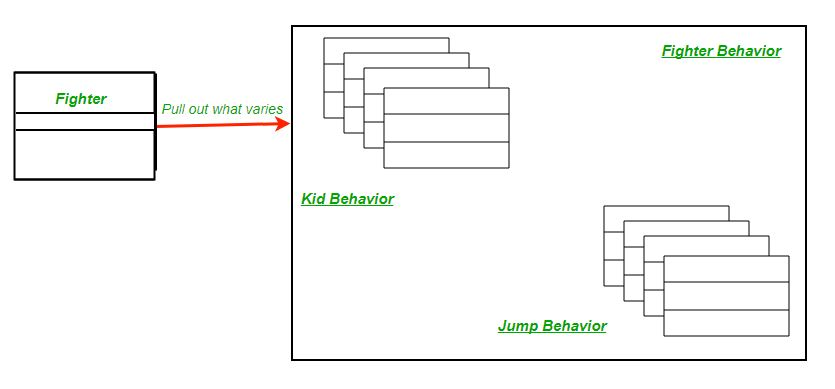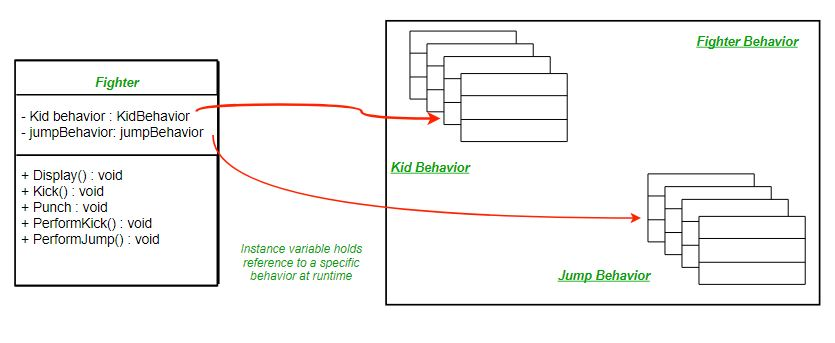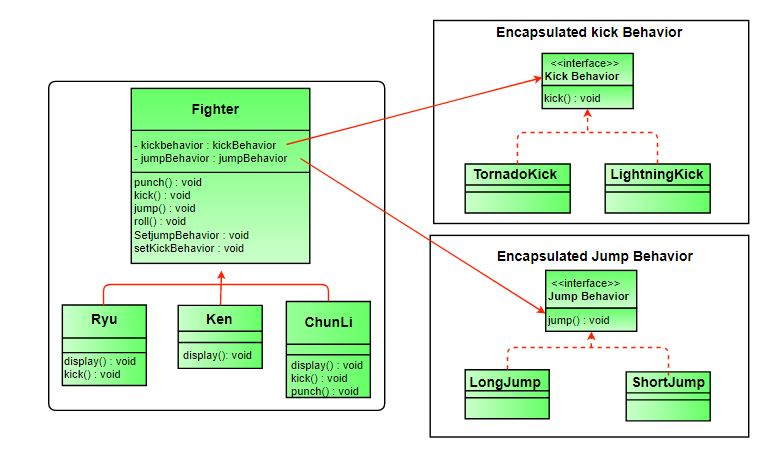Related Articles
Strategy Pattern | Set 2 (Implementation)
• Difficulty Level : Easy
• Last Updated : 14 Feb, 2018

We have discussed a fighter example and introduced Strategy Pattern in set 1.

Strategy Pattern | Set 1 (Introduction)

In this post, we apply Strategy Pattern to the Fighter Problem and discuss implementation.

The first step is to identify the behaviors that may vary across different classes in future and separate them from the rest. For our example let them be kick and jump behaviors. To separate these behaviors we will pull both methods out of Fighter class and create a new set of classes to represent each behavior.The Fighter class will now delegate its kick and jump behavior instead of using kick and jump methods defined in the Fighter class or its subclass.After reworking the final class diagram would be (Click on image for better view):Comparing our design to the definition of strategy pattern encapsulated kick and jump behaviors are two families of algorithms. And these algorithms are interchangeable as evident in implementation.

Below is the Java implementation of the same.

 `// Java program to demonstrate implementation of ` `// Strategy Pattern ` ` `  `// Abstract as you must have a specific fighter ` `abstract` `class` `Fighter ` `{ ` `    ``KickBehavior kickBehavior; ` `    ``JumpBehavior jumpBehavior; ` ` `  `    ``public` `Fighter(KickBehavior kickBehavior, ` `                   ``JumpBehavior jumpBehavior) ` `    ``{ ` `        ``this``.jumpBehavior = jumpBehavior; ` `        ``this``.kickBehavior = kickBehavior; ` `    ``} ` `    ``public` `void` `punch() ` `    ``{ ` `        ``System.out.println(``"Default Punch"``); ` `    ``} ` `    ``public` `void` `kick() ` `    ``{ ` `        ``// delegate to kick behavior ` `        ``kickBehavior.kick(); ` `    ``} ` `    ``public` `void` `jump() ` `    ``{ ` ` `  `        ``// delegate to jump behavior ` `        ``jumpBehavior.jump(); ` `    ``} ` `    ``public` `void` `roll() ` `    ``{ ` `        ``System.out.println(``"Default Roll"``); ` `    ``} ` `    ``public` `void` `setKickBehavior(KickBehavior kickBehavior) ` `    ``{ ` `        ``this``.kickBehavior = kickBehavior; ` `    ``} ` `    ``public` `void` `setJumpBehavior(JumpBehavior jumpBehavior) ` `    ``{ ` `        ``this``.jumpBehavior = jumpBehavior; ` `    ``} ` `    ``public` `abstract` `void` `display(); ` `} ` ` `  `// Encapsulated kick behaviors ` `interface` `KickBehavior ` `{ ` `    ``public` `void` `kick(); ` `} ` `class` `LightningKick ``implements` `KickBehavior ` `{ ` `    ``public` `void` `kick() ` `    ``{ ` `        ``System.out.println(``"Lightning Kick"``); ` `    ``} ` `} ` `class` `TornadoKick ``implements` `KickBehavior ` `{ ` `    ``public` `void` `kick() ` `    ``{ ` `        ``System.out.println(``"Tornado Kick"``); ` `    ``} ` `} ` ` `  `// Encapsulated jump behaviors ` `interface` `JumpBehavior ` `{ ` `    ``public` `void` `jump(); ` `} ` `class` `ShortJump ``implements` `JumpBehavior ` `{ ` `    ``public` `void` `jump() ` `    ``{ ` `        ``System.out.println(``"Short Jump"``); ` `    ``} ` `} ` `class` `LongJump ``implements` `JumpBehavior ` `{ ` `    ``public` `void` `jump() ` `    ``{ ` `        ``System.out.println(``"Long Jump"``); ` `    ``} ` `} ` ` `  `// Characters ` `class` `Ryu ``extends` `Fighter ` `{ ` `    ``public` `Ryu(KickBehavior kickBehavior, ` `               ``JumpBehavior jumpBehavior) ` `    ``{ ` `        ``super``(kickBehavior,jumpBehavior); ` `    ``} ` `    ``public` `void` `display() ` `    ``{ ` `        ``System.out.println(``"Ryu"``); ` `    ``} ` `} ` `class` `Ken ``extends` `Fighter ` `{ ` `    ``public` `Ken(KickBehavior kickBehavior, ` `               ``JumpBehavior jumpBehavior) ` `    ``{ ` `        ``super``(kickBehavior,jumpBehavior); ` `    ``} ` `    ``public` `void` `display() ` `    ``{ ` `        ``System.out.println(``"Ken"``); ` `    ``} ` `} ` `class` `ChunLi ``extends` `Fighter ` `{ ` `    ``public` `ChunLi(KickBehavior kickBehavior, ` `                  ``JumpBehavior jumpBehavior) ` `    ``{ ` `        ``super``(kickBehavior,jumpBehavior); ` `    ``} ` `    ``public` `void` `display() ` `    ``{ ` `        ``System.out.println(``"ChunLi"``); ` `    ``} ` `} ` ` `  `// Driver class ` `class` `StreetFighter ` `{ ` `    ``public` `static` `void` `main(String args[]) ` `    ``{ ` `        ``// let us make some behaviors first ` `        ``JumpBehavior shortJump = ``new` `ShortJump(); ` `        ``JumpBehavior LongJump = ``new` `LongJump(); ` `        ``KickBehavior tornadoKick = ``new` `TornadoKick(); ` ` `  `        ``// Make a fighter with desired behaviors ` `        ``Fighter ken = ``new` `Ken(tornadoKick,shortJump); ` `        ``ken.display(); ` ` `  `        ``// Test behaviors ` `        ``ken.punch(); ` `        ``ken.kick(); ` `        ``ken.jump(); ` ` `  `        ``// Change behavior dynamically (algorithms are ` `        ``// interchangeable) ` `        ``ken.setJumpBehavior(LongJump); ` `        ``ken.jump(); ` `    ``} ` `} `

Output :

```Ken
Default Punch
Short Jump
Long Jump
```

References: